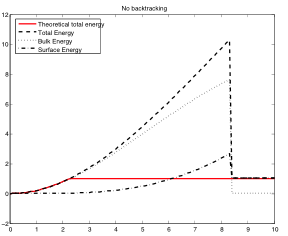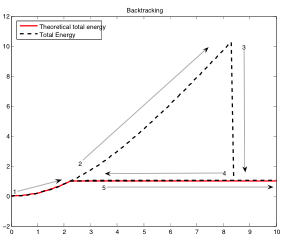# The Backtracking Algorithm

How to avoid some local minimizers and ensure energy balance.

One of the issues in the numerical implementation of Francfort and Marigo’s energy lies in its non-convexity. Indeed, this energy (and the regularizations used in its implementation) can sometimes possess many local minimizers, in which the numerical method might get trapped. Because $$\Gamma$$-convergence preserve only global and some local minimizers, local minimizers of the regularized energy may have little physical relevance.

The goal of the backtracking algorithm (Bourdin, 2007) is to detect a type of local minima frequently encountered in numerical experiments, using the crack growth condition and the form of the elastic potential. Considering a family of monotonically increasing loads, it is easy to show that for any two load increments $$s \le t$$, the deformation fields $$u_s$$, $$u_t$$ and crack sets $$K_s$$, $$K_t$$ must satisfy the condition

$\frac{s^2}{t^2} E_b(u_t) + E_s(K_t) \ge E_b(u_s) + E_s(K_s)$

where $$E_b$$ and $$E_s$$ correspond to the bulk and surface part of the fracture energy, respectively.

If this condition is not met, then $$(u_s,K_s)$$ cannot be a global minimizer of the fracture energy. In the Backtracking Algorithm, if such a violation is detected, one returns to load increment $$s$$, and initializes the minimization algorithm with $$(u_t,K_t)$$ built from $$(u_s,K_s)$$.

The following figures illustrate the Backtracking on a simple traction experiment on an elongated beam. For this problem, it is possible to show that there exists a critical load increment below which the optimal configuration correspond to an un-cracked domain. Above this threshold, any configuration with a transverse crack is a global minimizer. The leftmost figure represent a typical energy evolution. The red line represents the theoretical energy, the dashed line the numerical total energy. For loads in the 2.5-8.5 range, the numerical scheme fails to bifurcate towards the cracked solution and converges to a local minimizer that violates the condition above. The rightmost figure illustrates the outcome of the backtracking algorithm on this example. At first (steps 1, 2 in the figure), the algorithm converges to local minimizers. When it eventually bifurcates towards the cracked solution, (step 3) a violation of the backtracking condition is detected and the algorithm returns to the critical load increment. As the load is incremented, the algorithm converges now to the proper solution (step 5).Backtracking algorithm applied to a uniaxial tension experiment from (Bourdin, 2007).

1. Bourdin, B. (2007). Numerical implementation of a variational formulation of quasi-static brittle fracture. Interfaces Free Bound., 9, 411–430. DOI:10.4171/IFB/171 Download